# CSS Font (2) - 像素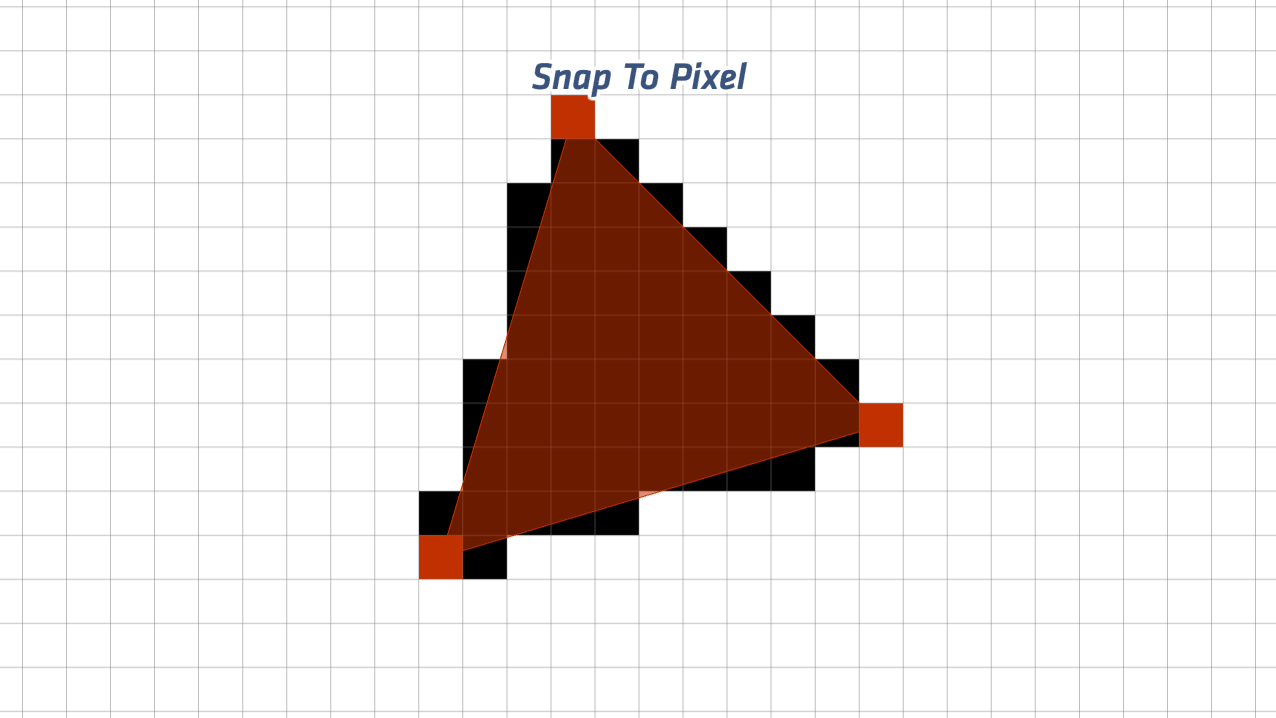（圖片取自 unconed 網站，版權為原網站所有。）

## Pixel

Full HD 的螢幕解析度是 1920x1080，表示螢幕橫向 X 軸有 1920 個像素單位，縱向 Y 軸有 1080 個像素單位，總共超過 200 萬個像素單位，這種螢幕實際所擁有的像素數量通常稱為裝置像素（device pixel）。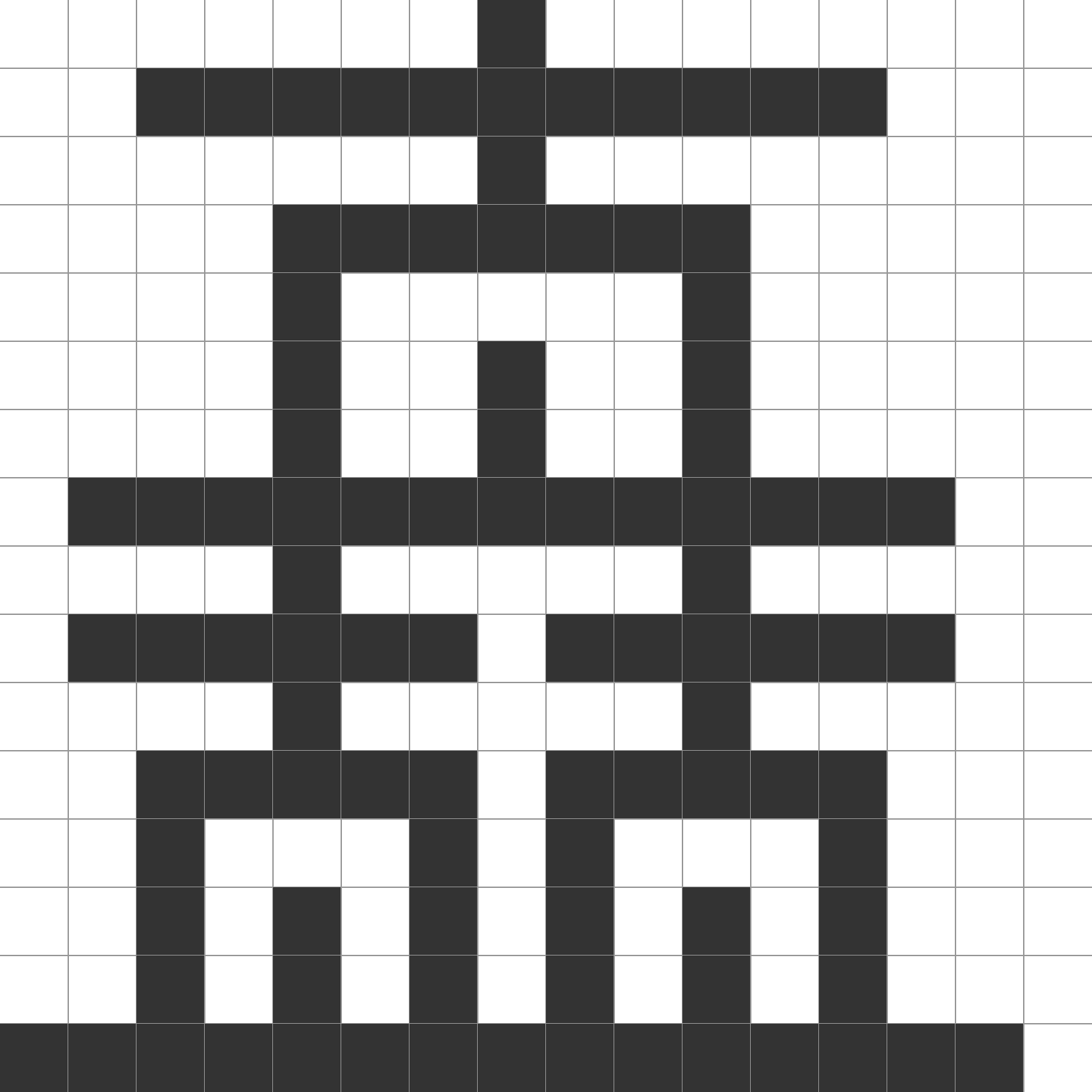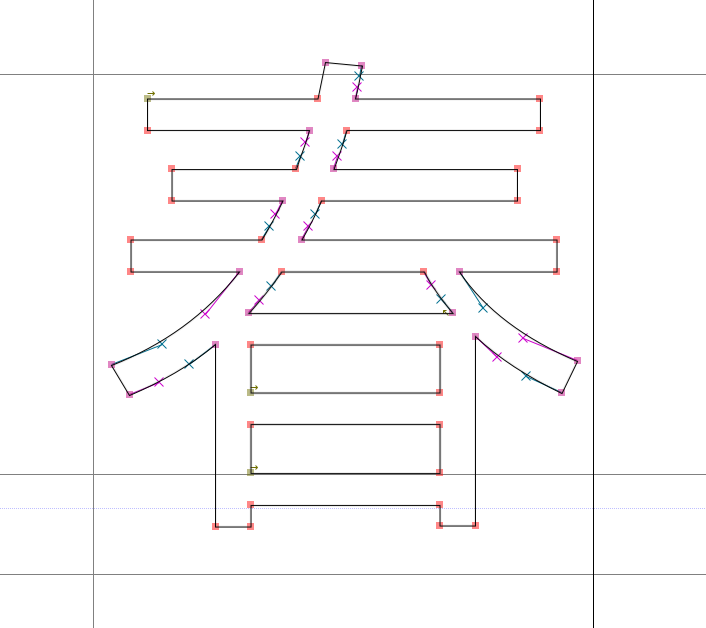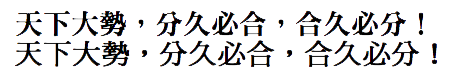## px 不是 Pixel

``````1in = 2.54cm = 25.4mm = 72pt = 6pc
``````

W3 早在 CSS Level 1 就提出了參考像素（reference pixel）的概念，定義了 CSS 的 px 單位是參考像素而不是裝置像素。參考像素 1px 等於 1/96 英吋（大約 0.26mm）。與其他絕對單位的轉換公式可以寫成：

``````1in = 96px = 72pt = 25.4mm
``````

``````12 / 72 * 96 = 16
``````

## 字形座標轉換 Pixel

``````x=474 y=0
x=137 y=0
x=137 y=1456
x=474 y=1456
``````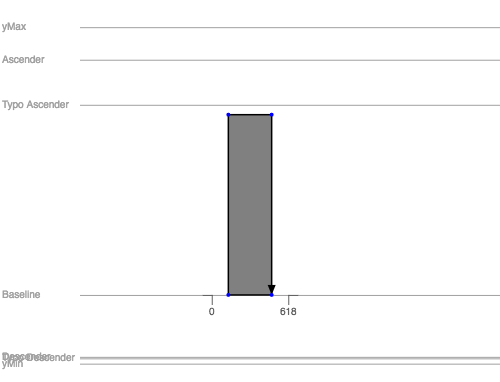``````(round(x/2048*16), round(y/2048*16))
``````

``````(3.7, 0)
(1.07, 0)
(1.07, 11.38)
(3.7, 11.38)
``````

``````X/Y軸使用的FUnit * ((字級大小pt * PPI / 72 * 字型UPM尺寸))
``````

``````337 * ((12 * 96) / (72 * 2048)) = 2.63
1456 * ((12 * 96) / (72 * 2048)) = 11.38
``````